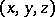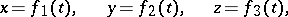# Reference system

(diff) ← Older revision | Latest revision (diff) | Newer revision → (diff)
Jump to: navigation, search

A-tuple consisting of a coordinate system and a clock connected to a body, in relation to which the motion (or equilibrium) of any other material points, or bodies is studied. Any motion is relative, and the motion of a body can only be considered in relation to some other body (reference body) or system of bodies. For example, it is impossible to describe the motion of the moon except in relation to the Earth, the Sun or the stars.

In mathematical terms, the motion of a body (or of a material point) in relation to a chosen reference system is described by equations which establish how the coordinates of the position of the body (or point) in this reference system change in the course of time. For example, in Cartesian coordinatesthe motion of a point is defined by the equationswhich are called equations of motion.

The choice of a reference system depends on the aim of the research. In kinematic research, all reference systems are equally applicable. In problems of dynamics, inertial systems (cf. Inertial system) are of paramount importance; in relation to these systems, differential equations usually take a simpler form.

#### Comments

How to Cite This Entry:
Reference system. Encyclopedia of Mathematics. URL: http://encyclopediaofmath.org/index.php?title=Reference_system&oldid=17040
This article was adapted from an original article by Material from the article "Reference system" in BSE-3 (originator), which appeared in Encyclopedia of Mathematics - ISBN 1402006098. See original article